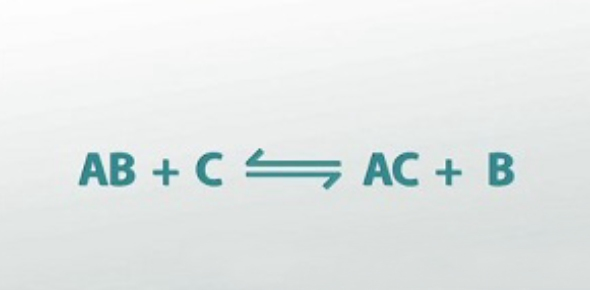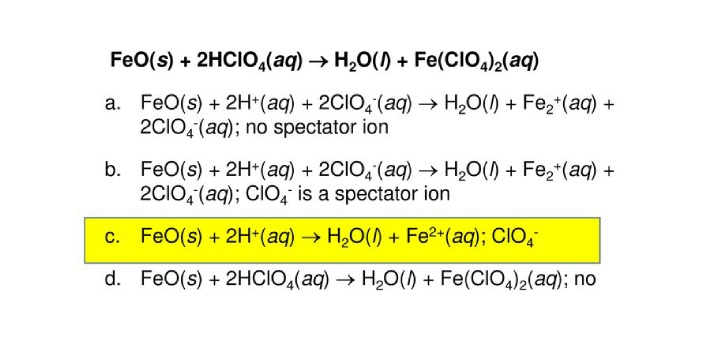What is the balanced net ionic equation for the following reaction? - ProProfs DiscussTopicsMoreProductsMore+ Ask Question

# What is the balanced net ionic equation for the following reaction? Identify the spectator ions, if any.Change Image    Delete

FeO(s) + 2HClO4(aq) --> H2O(l) + FE(ClO4)2(aq)

A. FeO(s) + 2H+(aq) ---> H2O(l) + Fe2+(aq); ClO4-, H+, and Fe2+ spectator ions
B. FeO(s) + 2H+(aq) ---> H2O(l) + Fe2+(aq); ClO4- spectator ion
C. FeO(s) + 2H+(aq) + 2ClO4-(aq) --> H2O(l) + Fe2+(aq) + 2ClO4-(aq); ClO4- spectator ion
D. FeO(s) + 2H+(aq) + 2ClO4-(aq) -> H2O(l) + Fe2+(aq) + 2ClO4-(aq); no spectator ions
E. FeO(s) + 2HClO4(aq) ---> H2O(l) + FE(ClO4)2(aq); no spectator ions

This question is part of Solution Chemistry Test-2
Asked by Sam, Last updated: Apr 01, 2020

###Request 0FollowShareAnswer AnonymouslyAnswer LaterCopy Link#### c.Dorothy

C.DorothyThe correct answer is FeO(s) + 2H (aq) → H2O(l) + Fe2(aq), with CIO4- as a spectator ion. For those who haven’t studied chemistry past what is offered in most American high schools, a spectator ion is pretty cool. The ion goes through the reaction, and comes out without a scratch. Therefore, it remains unchanged in the equation, and is often placed to the side of the final equation. In this instance, CIO4- is the spectator ion in our equation.As for getting the balanced net ionic equation, this is just another way to say that the equation has been balanced, and it shows the states of each ion. So, “s” would be a solid, “aq” would be aqueous, and “l” would be a liquid.#### ameier

Ameier

FeO(s) + 2H+(aq) ---> H2O(l) + Fe2+(aq); ClO4- spectator ionSearch for Google imagesSelect a recommended image
Upload from your computerCancelSearch for Google imagesSelect a recommended image
Upload from your computerCancelSearch for Google imagesSelect a recommended image
Upload from your computerCancel